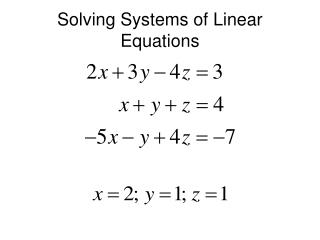DownloadDownload PresentationSolving Systems of Linear Equations

# Solving Systems of Linear Equations

Download Presentation## Solving Systems of Linear Equations

- - - - - - - - - - - - - - - - - - - - - - - - - - - E N D - - - - - - - - - - - - - - - - - - - - - - - - - - -
##### Presentation Transcript

1. Solving Systems of Linear Equations

2. Enter the Coefficients and Constants from the Equations

3. In an empty cell, type =minverse(data range), Enter

4. Press F2, then Ctrl+Shift+Enter

5. In a blank cell, type =mmult(range1,range2), Enter

6. Select a range containing the first cell that contains the same number of cells as unknowns

7. Press F2, Ctrl+Shift+Enter

8. Benefits of Matrix Method • Coefficients and Constants can be changed and the solutions are immediately calculated • Applies easily to large systems of equations. • Shortfalls: • Cannot be used for non-linear equations

9. Benefits of Solver Method • Can be used for all non-linear equations • Shortfalls: • Tedious for large systems of equations. • Initial guesses must be given, and solution may not converge if the guesses are not close to the actual solution. • Any change in the problem requires that Solver be rerun again.

10. Temperature Distribution in a Square Plate 100 oC 50 oC 75 oC 25 oC

11. j i Solution

12. 100 oC T11 T12 T13 T21 T22 T23 50 oC 75 oC T31 T33 T32 25 oC